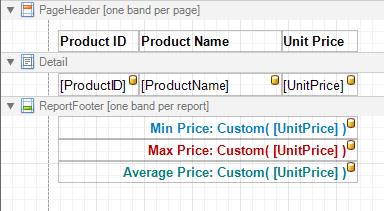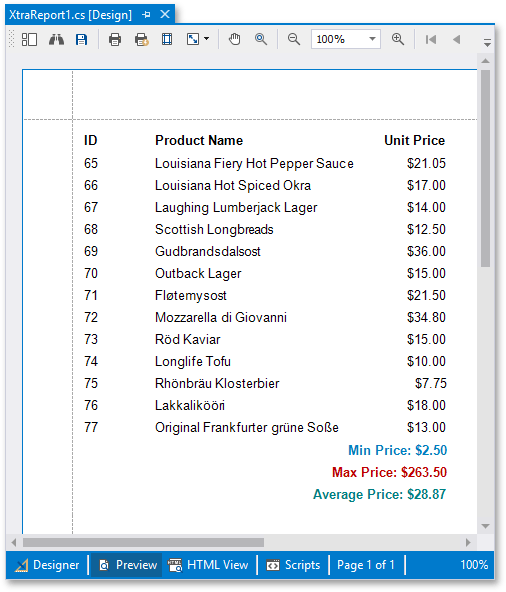# Handle Script Events to Calculate Custom Summaries

This example illustrates a legacy approach to evaluate a custom summary over a report’s data.

Important

Report scripts are not secure. We recommend that you use expression bindings instead.

Note

With Expressions Bindings, calculation of custom summaries is no longer available. You are encouraged to write a custom expression with operators, functions, and constants, as described in the following help topic: Expression Language. For additional examples refer to the following help section: Calculate Summaries.

In this example, a report is bound to the “Products” table (from the Northwind Traders demo database shipped with XtraReports). The following image illustrates this report’s design:The following are the scripts used to calculate the minimum value for the UnitPrice data field in the Products table of the Northwind database. Note that for this example to work correctly, xrLabel1 should be bound to the UnitPrice field, its XRSummary.Running property should be set to Report, and its XRSummary.Func property should be set to Custom. Then, switch to the Scripts tab, and handle the following script events of this label.

``````System.Decimal minPrice = System.Decimal.MaxValue;

private void OnSummaryReset(object sender, System.EventArgs e) {
minPrice = System.Decimal.MaxValue;
}

private void OnSummaryRowChanged(object sender, System.EventArgs e) {
minPrice = Math.Min(minPrice, (System.Decimal)GetCurrentColumnValue("UnitPrice"));
}

private void OnSummaryGetResult(object sender, SummaryGetResultEventArgs e) {
e.Result = minPrice;
e.Handled = true;
}
``````

The following are the scripts used to calculate the maximum value for the UnitPrice data field. Note that for this example to work correctly, xrLabel2 should be bound to the UnitPrice field, its XRSummary.Running property should be set to Report, and its XRSummary.Func property should be set to Custom. Then, switch to the Scripts tab, and handle the following script events of this label.

``````System.Decimal maxPrice = System.Decimal.MinValue;

private void OnSummaryReset(object sender, System.EventArgs e) {
maxPrice = System.Decimal.MinValue;
}

private void OnSummaryRowChanged(object sender, System.EventArgs e) {
maxPrice = Math.Max(maxPrice, (System.Decimal)GetCurrentColumnValue("UnitPrice"));
}

private void OnSummaryGetResult(object sender, SummaryGetResultEventArgs e) {
e.Result = maxPrice;
e.Handled = true;
}
``````

The following are the scripts used to calculate the average value for the UnitPrice data field. Note that for this example to work correctly, xrLabel3 should be bound to the UnitPrice field, its XRSummary.Running property should be set to Report, and its XRSummary.Func property should be set to Custom. Then, switch to the Scripts tab, and handle the following script events of this label.

``````System.Decimal totalPrice = 0;
int productsCount = 0;

private void OnSummaryReset(object sender, System.EventArgs e) {
totalPrice = 0;
productsCount = 0;
}

private void OnSummaryRowChanged(object sender, System.EventArgs e) {
productsCount++;
totalPrice += (System.Decimal)GetCurrentColumnValue("UnitPrice");
}

private void OnSummaryGetResult(object sender, SummaryGetResultEventArgs e) {
e.Result = productsCount > 0 ? totalPrice / productsCount : 0;
e.Handled = true;
}
``````

The following image illustrates the result: